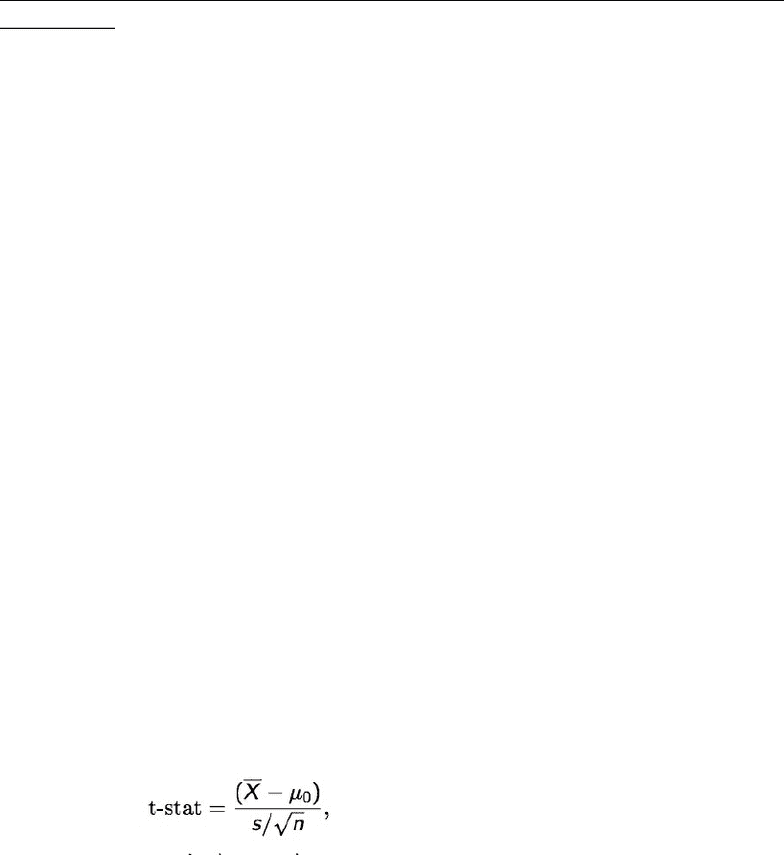Class Notes (1,100,000)
US (480,000)
UPenn (2,000)
STAT (70)
Lecture 19

# STAT 101 Lecture Notes - Lecture 19: Null Hypothesis, Test Statistic, Sample Size DeterminationPremium

Department
Statistics
Course Code
STAT 101
Professor
Richard Waterman
Lecture
19

This preview shows page 1. to view the full 4 pages of the document.Stat 101 - Introduction to Business Statistics - Lecture 19: Hypothesis Testing
(continued)
Summary of Testing Process
Set up the appropriate null and alternative hypothesis
identify the right test statistic
calculate the test statistic
compare the test statistic to the cut off value or compare the pvalue to alpha
The cut-off value comes from looking up the appropriate quantile in the t-tables,
but we often round this value to 2 for simplicity when the test is two-sided and α =
0.05.
if the test statistic exceeds the cut off value or the pvalue is less than alpha, then reject
the null hypothesis
otherwise, fail to reject the null
Significance Level
if we use a cut-off value of 2 for the test, then given the null is true there is only a 5%
chance of making a type I error
We call this 5% a significance level and write it as α = 0.05
likewise, if the cutoff value was 1.645 then α = 0.10
One-Sample T-Test for the population Mean
The test statistic for testing a single mean against a hypothesized value is called the t-
test statistic
The test is defined as
n = sample size
s = sample standard deviation
Xbar = sample mean
µ0 = hypothesized value
On observing a rare event - ie. Xbar being far from µ0 - we will doubt
assumptions under which it is defined to be rare and thus reject the null
hypothesis
In JMP..